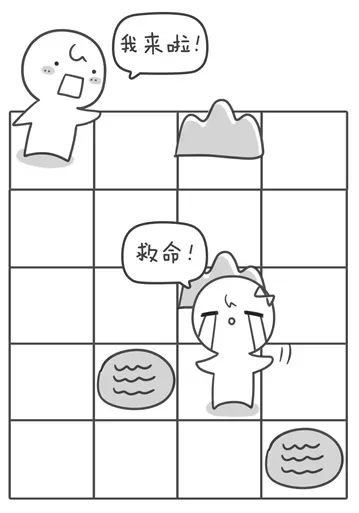• 累计撰写 144 篇文章
• 累计创建 26 个标签
• 累计收到 44 条评论

### 目 录CONTENT# 解救小哈——广度优先搜索2022-05-06 / 0 评论 / 0 点赞 / 228 阅读 / 721 字 / 正在检测是否收录...

# 解救小哈——广度优先搜索5 4
0 0 1 0
0 0 0 0
0 0 1 0
0 1 0 0
0 0 0 1
1 1 4 3


#include<stdio.h>
struct note {
int x; //横坐标
int y; //纵坐标
int s; //步数
};

int main(){
struct note que; //因为地图大小不超过50*50，因此队列拓展不会 超过2500个

int a = {0}, book = {0};

//定义一个用于表示走的方向的数组
int next = {{0, 1},{1, 0},{0, -1},{-1, 0}};

int i, j, k, n, m, startx, starty, tx, ty, flag, p, q;

scanf("%d %d",&n, &m);
for (i = 1 ; i <= n ; i ++)
for (j = 1 ; j <= m ; j ++)
scanf("%d",&a[i][j]);
scanf("%d %d %d %d",&startx, &starty, &p, &q);

//队列初始化
tail = 1;
//往队列插入迷宫入口坐标
que[tail].x = startx;
que[tail].y = starty;
que[tail].s = 0;
tail++;
book[startx][starty] = 1;

flag = 0;//用来标记是否打到目标点，0表示暂时还没有到达，1表达到达
//当队列不为空的时候循环
//枚举四个方向
for (k = 0 ; k <= 3 ; k ++){

//判断越界
if (tx < 1 || tx > n || ty < 1 || ty > m){
continue;
}
//判断是否是障碍物或者已经在路径中
if (a[tx][ty] == 0 && book[tx][ty] == 0){
//把这个点标记为已经走过
//宽搜每个点只入队一次，所以和深搜不同，不需要将book数 组还原
book[tx][ty] = 1;
//插入新的点到队列
que[tail].x = tx;
que[tail].y = ty;

que[tail].s = que[head].s + 1; //步数是父亲的步数 + 1
tail++;
}
//如果找到目标点了，就停止扩展，任务结束，退出循环
if (tx == p && ty == q){
flag = 1;
break;
}
}
if (flag == 1){
break;
}